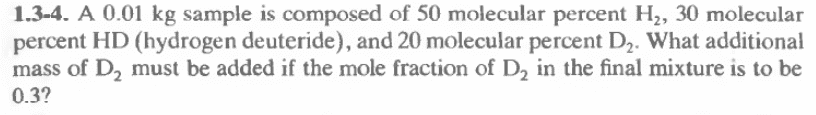# Molecular percent of compounds

• Chemistry
LCSphysicist
Homework Statement:
All below
Relevant Equations:
All belowmx remets to mass in gram of the compost x
nx remets to moles of the compost x

nTot remets to the total moles
x remets to the additional moles of D2
I considered the molar mass:
MH2 = 2u
MHD = 3u
MD2 = 4u

10 = mH2 + mHD + mD2
10 = nH2*2 + nHD*3 + nD2*4
10 = nTot + 0.9nTot + 0.8nTot
nTot = 10/2.7

So:
0.3 = (nD2 + x)/nTot + x
x = nTot/7
x = 10/(18.9)

That is, we need to add + 10/(18.9) moles of D2,
10/(18.9) = m/4
m = 2.1 g

This seems right?

Mentor
Homework Statement:: All below
Relevant Equations:: All below

View attachment 267794

mx remets to mass in gram of the compost x
nx remets to moles of the compost x

nTot remets to the total moles
x remets to the additional moles of D2
I considered the molar mass:
MH2 = 2u
MHD = 3u
MD2 = 4u

10 = mH2 + mHD + mD2
10 = nH2*2 + nHD*3 + nD2*4
10 = nTot + 0.9nTot + 0.8nTot
nTot = 10/2.7

So:
0.3 = (nD2 + x)/nTot + x
x = nTot/7
x = 10/(18.9)

That is, we need to add + 10/(18.9) moles of D2,
10/(18.9) = m/4
m = 2.1 g

This seems right?
That's what I get. Nice job.

•LCSphysicist